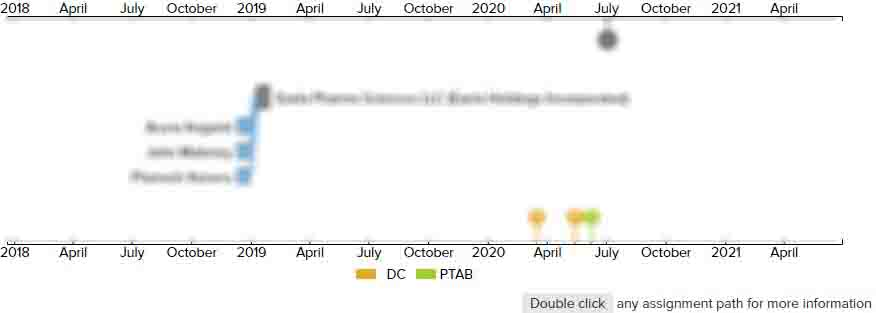# Micro inertial measurement unit

DC CAFC
• US 6,671,648 B2
• Filed: 10/22/2001
• Issued: 12/30/2003
• Est. Priority Date: 01/04/2000
• Status: Expired due to Term
##### First Claim
Patent Images

1. A micro inertial measurement unit, comprising:

• an angular rate producer comprising a X axis angular rate detecting unit which produces a X axis angular rate electrical signal, a Y axis angular rate detecting unit which produces a Y axis angular rate electrical signal, and a Z axis angular rate detecting unit which produces a Z axis angular rate electrical signal;

an acceleration producer comprising a X axis accelerometer which produces a X axis acceleration electrical signal, a Y axis accelerometer which produces a Y axis acceleration electrical signal, and a Z axis accelerometer which produces a Z axis acceleration electrical signal; and

an angular increment and velocity increment producer, which is electrically connected with said X axis, Y axis and Z axis angular rate detecting units and said X axis, Y axis and Z axis accelerometers, receiving said X axis, Y axis and Z axis angular rate electrical signals and said X axis, Y axis and Z axis acceleration electrical signals from said angular rate producer and said acceleration producer respectively, wherein said X axis, Y axis and Z axis angular rate electrical signals and said X axis, Y axis and Z axis acceleration electrical signals are converted into are digital angular increments and digital velocity increments respectively.

• ##### 1 Assignment
Timeline View
Assignment View×
×